### Keypoints#

The Keytopics in the blogpost:

• Presizing / Augmentation of Images
• Datablock
• Cross-Entropy Loss

#### Presizing / Augmentation of Images#

• The main idea behind augmenting the images is to reduce the number of computations and lossy operations. This also results in more efficient processing on the GPU.
• To make the above possible we need our images to have same dimensions, so they can be easily collated.
• Some of the challenges in doing the augmentation is that when we resize, the data could be degraded, new empty zones are introduced etc.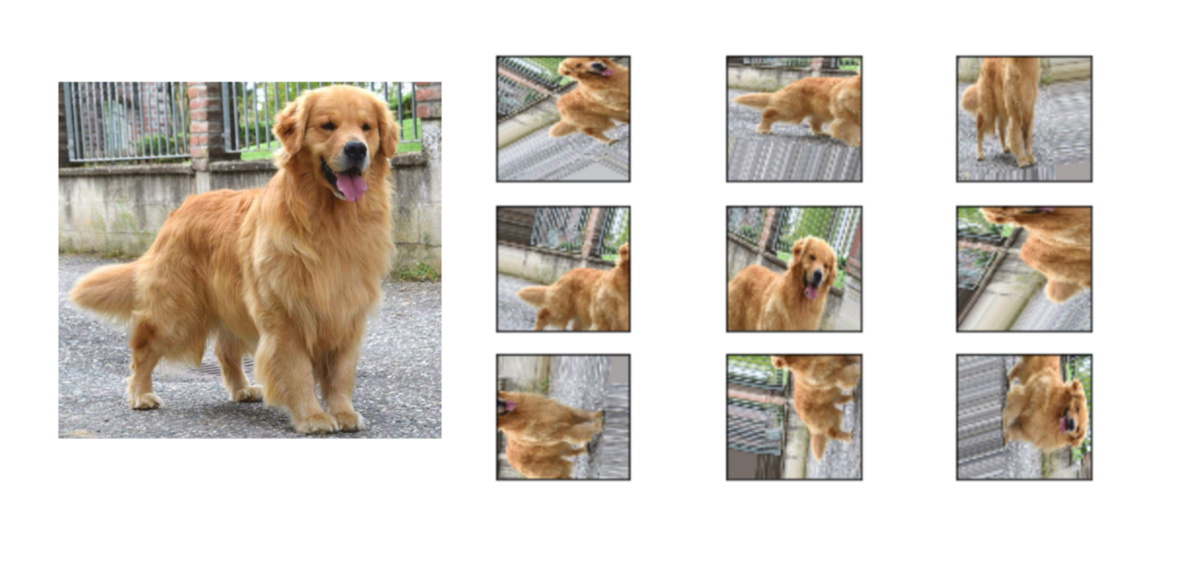Overcoming stratagies

• There are around two strategies:
• Resize images to relatively larger dimensions than the target training dimensions.

• Having all the augmentation operations done at once on the GPU at end of processing rather than performing operations individually and interpolating multiple times. -Two important things to note in the below example:

 ``````1 2 3 4 5 6 `````` ``````pets = DataBlock(blocks = (ImageBlock, CategoryBlock), get_items=get_image_files, splitter=RandomSplitter(seed=42), get_y=using_attr(RegexLabeller(r'(.+)_\d+.jpg\$'), 'name'), item_tfms=Resize(460), batch_tfms=aug_transforms(size=224,* min_scale=0.75)) ``````
• item_tmfs is applied to each individual image before its copied to GPU. And it ensures three things, that all images are the same size and on the training set, the crop area is chosen randomly and the validation set, the center square of the image is chosen.

• batch_tfms is applied to a batch all at once on the GPU.

#### Datablock#

• A datablock is a generic container to quickly build ‘Datasets’ and ‘DataLoaders’ .

• To build a datablock we need to know what kind of TransformBlock like a ImageBlock, CategoryBlock, which method to fetch the items like get_image_files , how to split the images, how to get the labels and any transformations to be applied.

• The below example repeated same as above in this context is a how we create a datablock:

 ``````1 2 3 4 5 6 `````` ``````pets = DataBlock(blocks = (ImageBlock, CategoryBlock), get_items=get_image_files, splitter=RandomSplitter(seed=42), get_y=using_attr(RegexLabeller(r'(.+)_\d+.jpg\$'), 'name'), item_tfms=Resize(460), batch_tfms=aug_transforms(size=224, min_scale=0.75)) ``````
• Once we have the datablock, we can get the dataloader object by just calling the dataloaders method on that datablock

 ``````1 `````` ``````dls = pets.dataloaders(path/"images") ``````
• So to ensure that we have the datablock created properly without any errors, we can do that with the following piece of code

 ``````1 `````` ``````dls.show_batch(nrows=1, ncols=3) ``````
• One of the important debugging method to learn when we have trouble creating a proper datablock is summary method. The summary method will provide very detailed stack trace

 ``````1 `````` ``````pets.summary(path/"images") ``````

#### Cross-Entropy Loss#

Cross-Entropy loss is use of negative loss on probabilities. Or in simple terms Cross-Entropy loss is a combination of using the negative log likelihood on the log values of the probabilities from the softmax function.

• The below image depicts the cross-entropy loss: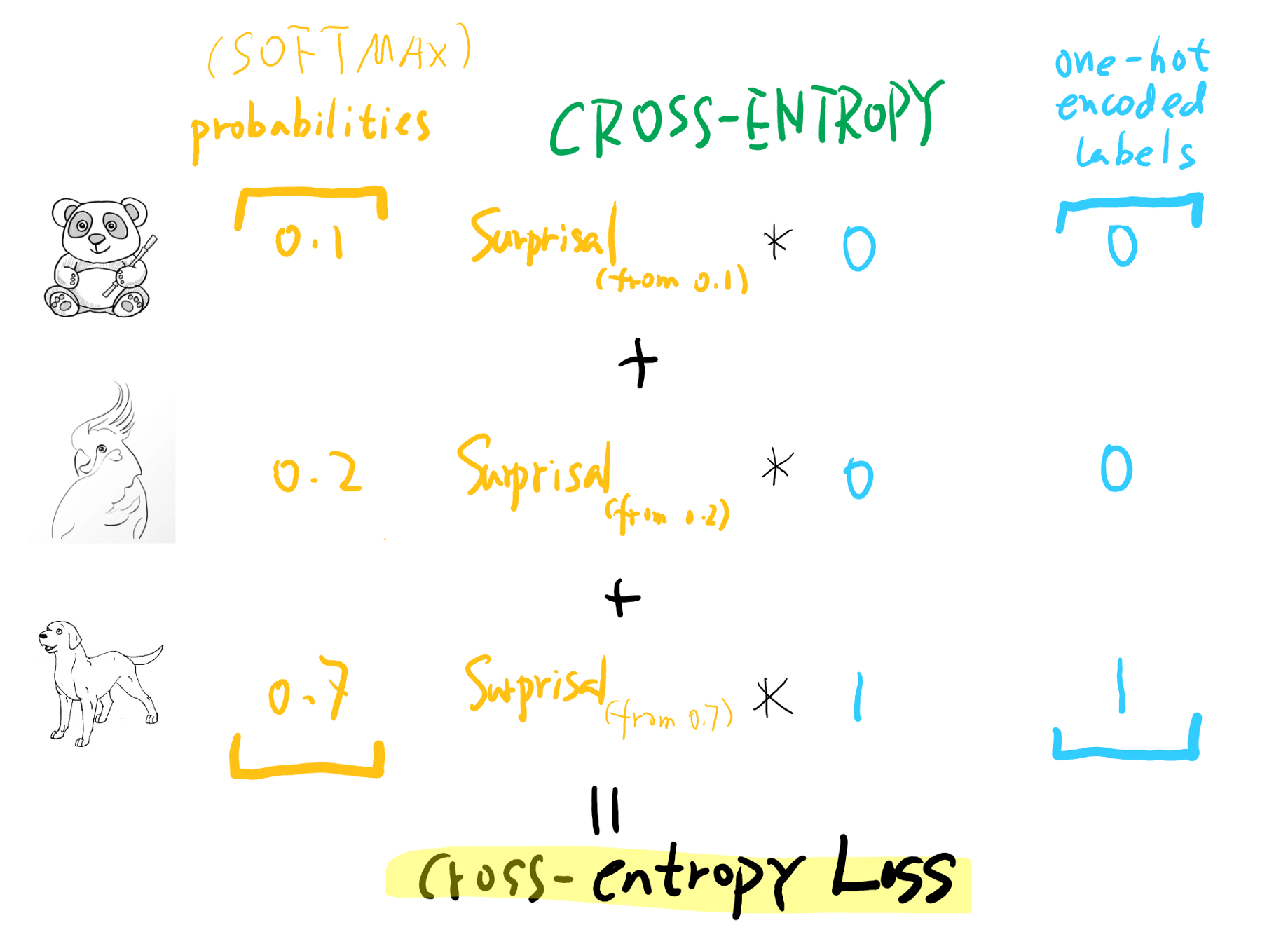• The best suited loss for the Image data and a categorical outcome is Cross-Entropy loss. When we haven’t provided the loss function we want to use, the fastAI by default will pick the cross-entropy.
• Cross-Entropy loss works even when with multi-categories of dependent variables.
• And this also results in faster and more reliable training.
• To transform the activations of our model into predictions, we use something called the softmax activation function.

Softmax Function:

• A softmax function will ensure that all the activations in the final layer of our classification model are between 0 and 1 and they all sum up to 1.
• It is more or less similar to sigmoid function.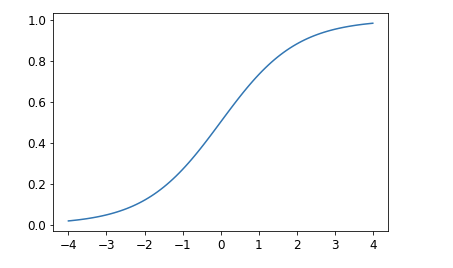• A sigmoid function when applied to single column of activations from neural network will return a column of numbers from 0 and 1. Now we are chosing softmax function since we have multi-categories and we need activations per category.
• If we are trying to apply the softmax function for two categories, it returns the same values as sigmoid for the first column and those subtracted from 1 for the second column.
 ``````1 2 `````` ``````def softmax(x): return exp(x) / exp(x).sum(dim=1, keepdim=True) ``````

Exponential function (exp) is defined as e**x, where e is a special number approximately equal to 2.718. It is the inverse of the natural logarithm function. Note that exp is always positive, and it increases very rapidly!

• We need exponential since it ensures that all numbers are positive and dividing by the sum ensures that they all add up to 1.
• And softmax function is better at picking one class among others, so it is ideal for training.
• The second part of the cross-entropy loss is Log Likelihood after the softmax function.

Log Likelihood:

• Lets consider an example of having 0.99 and 0.999 as probabilities they are very close but in terms of confidence the 0.999 is more confident than 0.99. So to transform the numbers between the negative infinity and 0 to 0 and 1.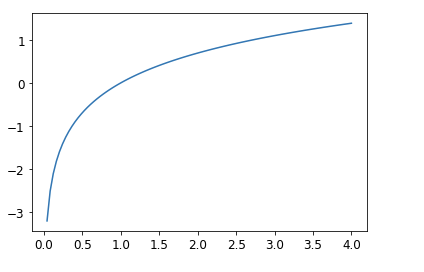• So taking the mean of the positive or negative log of our probabilities (depending on whether it’s the correct or incorrect class) gives us the negative log likelihood loss. In PyTorch, nll_loss assumes that you already took the log of the softmax, so it doesn’t actually do the logarithm for you.
• The CrossEntropyLoss function from pytorch exactly does the same: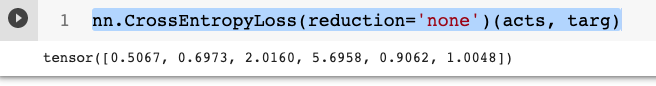### Making a Model better#

• How to interpret a model
• Learning Rate Finder
• Unfreezing & Transfer Learning

#### How to interpret a model#

• A usual way of interpreting or evaluating the model is looking at the metrics like a confusion matrix which will show where the model is performing poorly.
• But one of the toughest part of interpreting confusion matrix is when it has multi-category.
• We can overcome this by using a FastAI convenience function like most_confused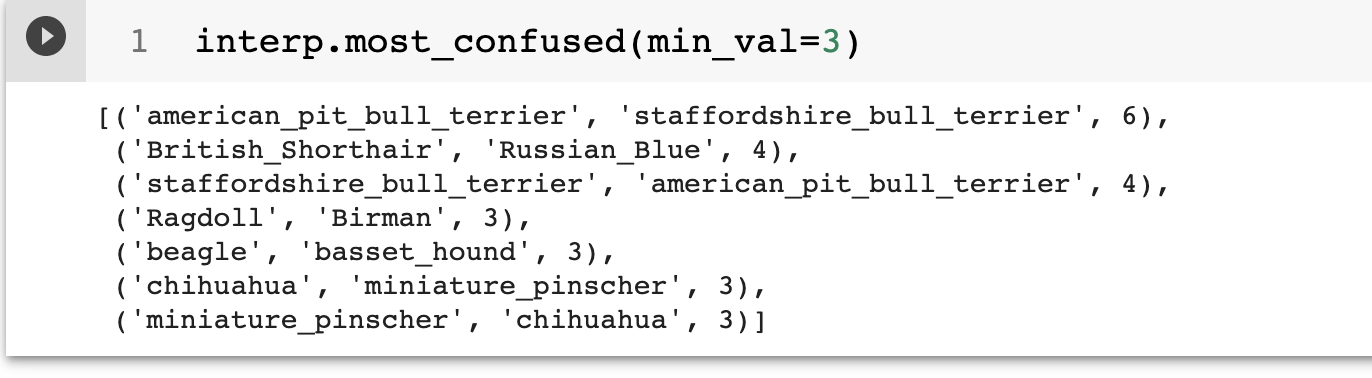#### Learning Rate Finder#

• One of the key points to consider when training a model would be to have a right learning rate and that can be found using the lr_find method, originally proposed by researcher Leslie Smith.
 ``````1 2 `````` ``````learn = cnn_learner(dls, resnet34, metrics=error_rate) lr_min,lr_steep = learn.lr_find() ``````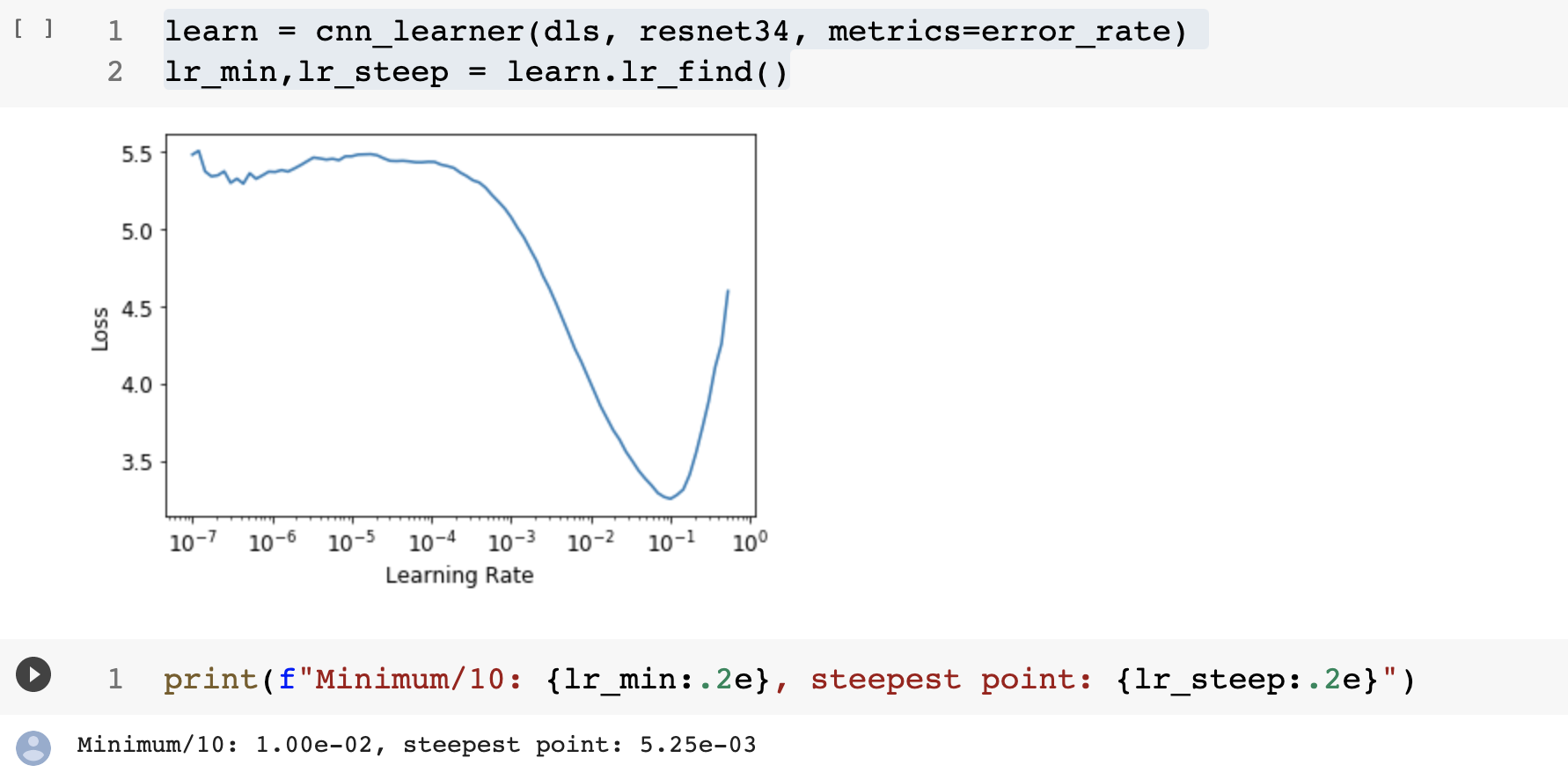#### Unfreezing & Transfer Learning#

• When training the model on a certain task, the optimizer should update the weights in the randomly added final layers. But we do not change the weights in the rest of the neural network at all. This is called freezing the pre-trained layers.
• When we are fine tuning the model, the fastai does two things:
• Trains the randomly added layers for one epoch, with all other layers frozen.
• Unfreezes all of the layers, and trains them all for the number of epochs requested.
• Instead of doing the fine-tuning from the library, we will also be able to do that manually. In that case we can unfreeze the layers by using the below code snippet.

#### Conclusion:#

• Cross-Entropy loss is for multi-category classification and is simply the use of negative loss on probabilities.
• To make our model better we can perform many steps right from data preparation which involves techniques like Pre-sizing to fine_tuning the model by fining proper learning rates. So each step has to be taken care in the whole process to yield better accuracy.